# USA Traveling Salesman Tour

Find the optimum traveling salesman tour through the capitals of the 48 contiguous states in the USA.

### Contents

#### State Capitals

I am continuing with the workspace from my previous blog post.

John Burkardt provides a second data set, state_capitals_ll.txt, giving the longitudes and latitudes of capitals of the 48 contiguous states. (Ignore AK, DC, HI, PR and US.)

    [lon,lat] = get_capital_coordinates;


When I use these coordinates for the graph I created in my previous post, we get a more accurate picture of the USA.

    G = graph(A,cellstr(names));
plot(G,'xdata',lon,'ydata',lat);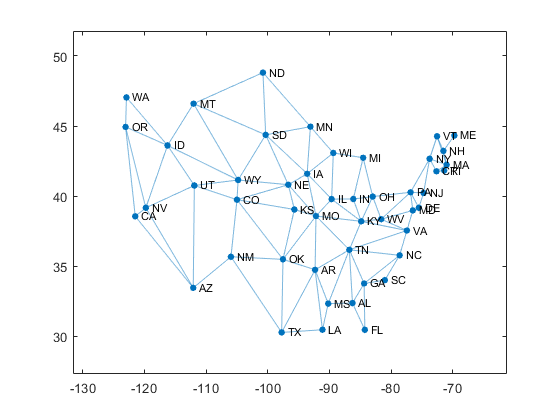#### Travel

The travelling salesman problem, the TSP, was mathematically formulated in the 19th century. The problem is to find the closed circuit of a list of cities that travels the shortest total distance.

For 25 years MATLAB releases have included a simple demo program named travel that finds an approximate solution to the TSP through a few dozen randomly chosen points. The background of the plot, an outline the USA, is just for artistic effect.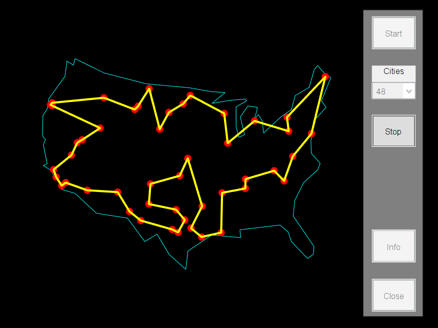#### Traveler

I've made the travel demo into a function named traveler whose input is the distance matrix D, the pairwise distances between the cities. The output is a path length miles and permutation vector p so that miles is the length of the route produced by lon(p) and lat(p).

Each time it is called, travel starts with a random permutation of the cities. Then, for several thousand iterations, it tries to reduce the length of the trip by revising the permutation in each of two simple ways. One scheme is to move one city to another position in the ordering. This example starts with the order [1:7]. It then moves city 4 to follow city 5, so the order becomes [1 2 3 5 4 6 7]. This reduces the length from 9.71 to 7.24.

   half_fig
traveler_scheme1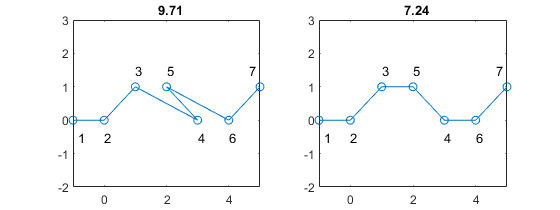The second scheme is based on the observation that any time a route crosses itself, the length can be reduced by reversing the crossed segment. We don't actually look for crossings, we just try reversing random chunks of the ordering. This example reverses the [3 4 5] in [1:7] to get [1 2 5 4 3 6 7], thereby reducing the length from 9.47 to 7.65.

   traveler_scheme2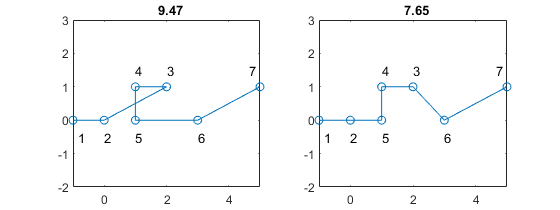These two heuristics are not powerful to guarantee that traveler will find the global shortest path. It's easy to get stuck in local minima. And the random path approach means that traveler is inefficient for more than a few dozen cities.

But nobody knows how to find a guaranteed shortest path for the TSP and the code for traveler is only 58 lines. I will include the code at the end of this post and in Cleve's Laboratory on the MATLAB Central File Exchange.

    close


#### Distance Matrix

The input to traveler is the distance matrix.

    D = distances(lon,lat);


The inputs to the function distances are the vectors lon and lat, the longitude and latitude of the cities. The output is the 48-by-48 matrix D, the great circle distance between pairs of cities. With some spherical trigonometry, lon and lat, which are essentially angles measured in degrees, are converted to miles, the lengths of "as the crow flies" geodesics on the surface of the earth. The radius of the earth is a scale factor. Here is the core of the function.

    dbtype 11:20 distances.m

11        R = 3959; % radius of the earth (mi.)
12        n = length(lon);
13        D = zeros(n,n);
14        for k = 1:n
15            for j = 1:k-1
16                D(k,j) = R*acos(sind(lat(k))*sind(lat(j)) + ...
17                    cosd(lat(k))*cosd(lat(j))*cosd(lon(k)-lon(j)));
18                D(j,k) = D(k,j);
19            end
20        end


#### Extrema

Which two capitals are nearest each other?

    Dmin = min(min(D(D>0)))
[k,j] = find(D == Dmin)
cities = names(k)

Dmin =
34.9187
k =
37
19
j =
19
37
cities =
2×1 string array
"RI"
"MA"


Providence, Rhode Island and Boston, Massachusetts ("our fair city") are less than 35 miles apart.

What about the other extreme?

    Dmax = max(max(D))
[k,j] = find(D == Dmax)
cities = names(k)

Dmax =
2.6629e+03
k =
17
4
j =
4
17
cities =
2×1 string array
"ME"
"CA"


As we might have expected, the capitals of Maine and California are the farthest apart, 2663 miles. That would require one prodigious crow.

Based on some experience that I will describe shortly, I am going to set the random number seed to 347 before we take our road trip with traveler.

    rng(347)
[miles,p] = traveler(D);
miles = round(miles)

miles =
10818


That's an average of

    avg = miles/48

avg =
225.3750


miles per leg.

#### Highlight

Let's highlight our graph of neighboring states with our traveling salesman path linking capital cities.

    Gp = plot(G,'xdata',lon,'ydata',lat,'edgecolor',turquoise);
highlight(Gp,p,'edgecolor',darkred,'linewidth',2)
title(miles)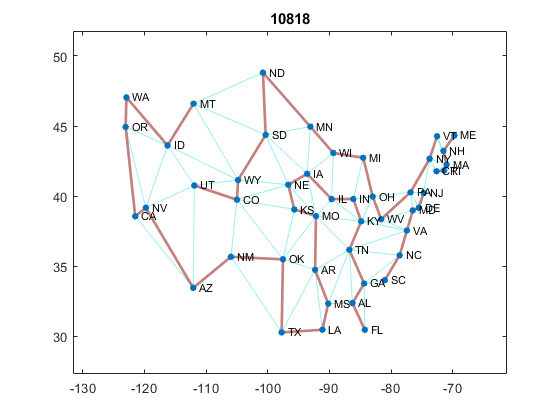There are four missing links. The path goes from UT to MT, but those states are not neighbors. We must go through ID. And the leg from FL to SC goes through GA. There are two more in the northeast. Fill in those missing links with dashed lines.

    missing_links(A,lon,lat,p,darkred)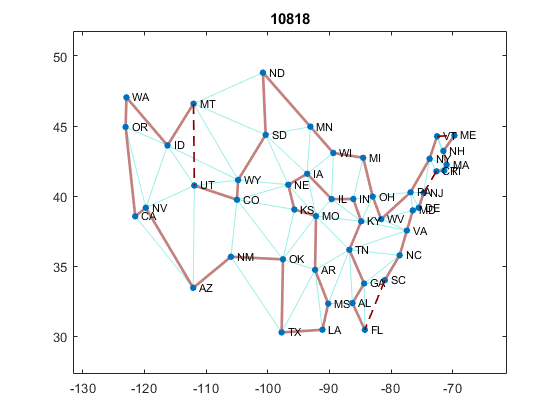Zoom in on the northeast.

    axis([-84 -68 33 46])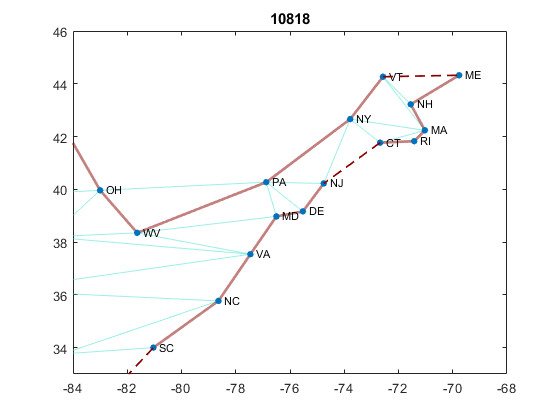#### Final Order

Here the state abbreviations, ordered by this path.

    fprintf(fmt,names(p))

LA TX OK NM AZ NV CA OR WA ID MT UT CO WY SD ND
MN WI MI OH WV PA NY VT ME NH MA RI CT NJ DE MD
VA NC SC FL AL GA TN KY IN IL IA NE KS MO AR MS
LA 

#### Last Step

It is interesting to look at the last step. After 3298 iterations, travel arrives at this situation with a path length of 10952.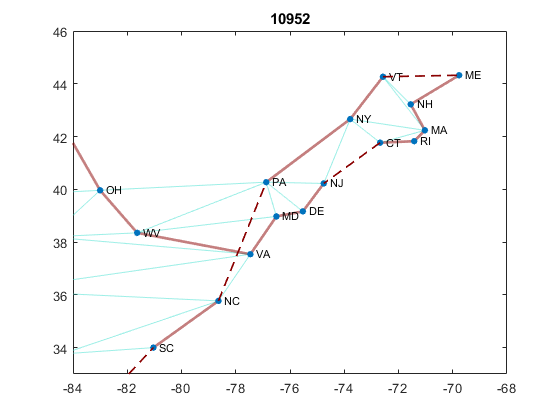The link connecting WV to VA crosses the link connecting NC to PA. It takes travel 222 more iterations, but eventually a permutation that reverses the path from VA and PA is tried. This connects WV to PA and NC to VA to produce the path shown in the previous plot. The total path length is decreased by 134 miles to 10818.

#### Optimum

I am confident that we have found the shortest path, but I don't have a proof.

I ran 1000 different initializations of rng. Eight runs produced the path with length 10818 that we found with rng(347). None of the runs found a shorter path. Sixteen more runs found a quite different path with length 10821, only three miles longer. Here is this next best path.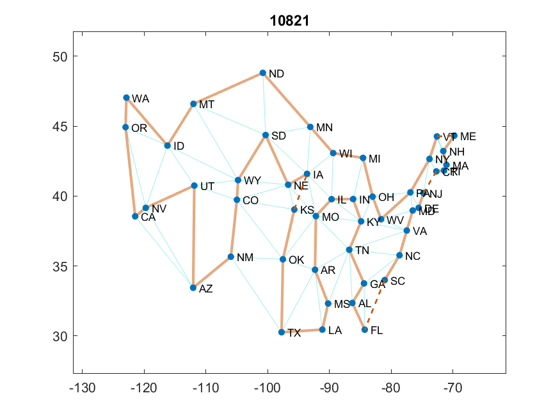#### Animation

I must include an animated gif here. This initially shows every sixth step. It switches to every step when the path length is less than 12000. Few legs in random paths match edges in the neighbor graph so initially most lines are dashed. As we near the minimum, more solid lines appear.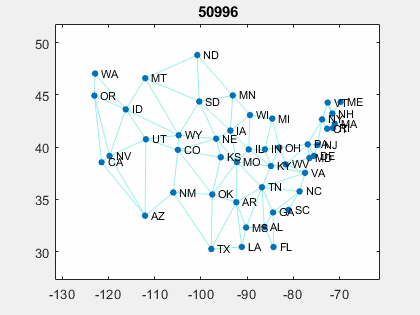#### Traveler_game

Chances that, without help, traveler will find the shortest route through the 48 capital cities are less than 1 in 100. Here is your opportunity to help guide the search. The traveler_game is a modification of travel that plots every successful step and that provides controls so that you to back up a few steps and try the random strategy again.

I will include the traveler_game in the next update, version 3.70, of Cleve's Laboratory on the MATLAB Central File Excange. That may take a few days.

There are five push buttons.

• > Take one minimization step.
• >> Take repeated steps, until a local minimum is reached.
• < Reverse one step.
• << Reverse many steps.
• ^ Start over in a new figure window.

Here are four typical runs.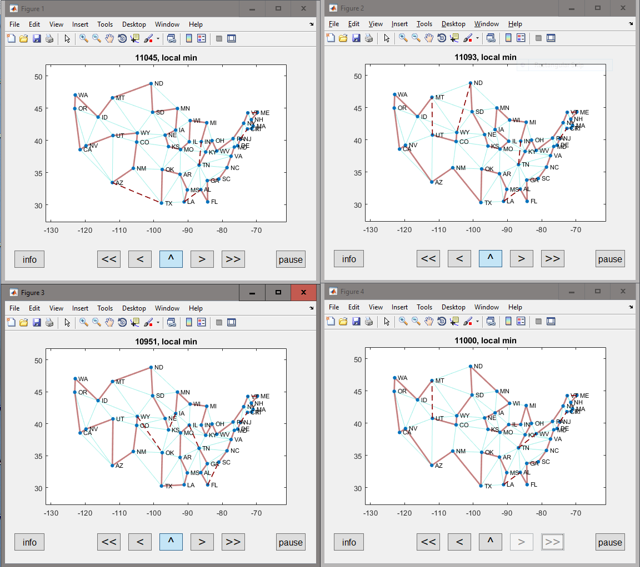#### Codes

Here are the codes for traveler, distances, and path_length.

    type traveler
type distances
type path_length

function [len,p] = traveler(D)
%TRAVELER  Functional form of old MATLAB demo, "travel".
%   A pretty good, but certainly not the best, solution of the
%   Traveling Salesman problem.  Form a closed circuit of a
%   number of cities that travels the shortest total distance.
%
%   [len,p] = traveler(D).
%   Input: D = distances between cities with coordinates x and y.
%   Output: p a permutation, x(p) and y(p) is a path with length len.

%   Copyright 1984-2018 The MathWorks, Inc.

n = size(D,1);
p = randperm(n);
len = path_length(p,D);

for iter = 1:10000

% Try to reverse a portion.

pt1 = floor(n*rand)+1;
pt2 = floor(n*rand)+1;

lo = min(pt1,pt2);
hi = max(pt1,pt2);

q = 1:n;
q(lo:hi) = q(hi:-1:lo);
pnew = p(q);

lennew = path_length(pnew,D);
if lennew < len
p = pnew;
len = lennew;
iterp = iter;
end

% Try a single point insertion

pt1 = floor(n*rand)+1;
pt2 = floor((n-1)*rand)+1;

q = 1:n;
q(pt1) = [];
q = [q(1:pt2) pt1 q((pt2+1):(n-1))];
pnew = p(q);

lennew = path_length(pnew,D);
if lennew < len
p = pnew;
len = lennew;
iterp = iter;
end
end

% Close the permutation.
p(end+1) = p(1);
end

function D = distances(lon,lat)
%   D = distances(lon,lay)
%   Input: vectors lon and lat, the longitude and latitude of the cities.
%   Output: D(k,j) is the distance (in miles) between cities k and j.

%   Copyright 1984-2018 The MathWorks, Inc.

% Great circle distance matrix between pairs of cities.
% https://en.wikipedia.org/wiki/Great-circle_distance.

R = 3959; % radius of the earth (mi.)
n = length(lon);
D = zeros(n,n);
for k = 1:n
for j = 1:k-1
D(k,j) = R*acos(sind(lat(k))*sind(lat(j)) + ...
cosd(lat(k))*cosd(lat(j))*cosd(lon(k)-lon(j)));
D(j,k) = D(k,j);
end
end
end

function len = path_length(p,D)
% len = path_length(p,D)

% Calculate current path length for traveling salesman problem.
% This function calculates the total length of the current path
% p in the traveling salesman problem.
%
% This is a vectorized distance calculation.
%
% Create two vectors: p and q = p([n 1:(n-1)]).
% The first is the list of first cities in any leg, and the second
% is the list of destination cities for that same leg.
% (the second vector is an element-shift-right version of the first)
%
% Use column indexing into D to create a vector of
% lengths of each leg which can then be summed.

n = length(p);
q = p([n 1:(n-1)]);
len = sum(D(q + (p-1)*n));

end


Published with MATLAB® R2018a

|